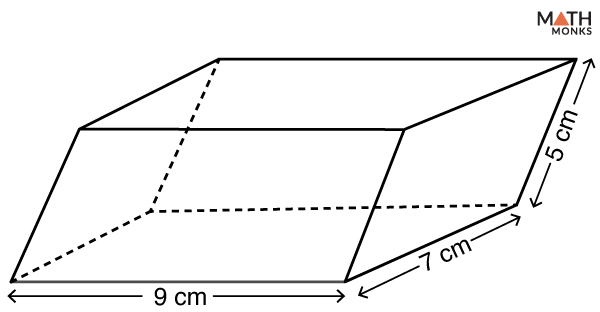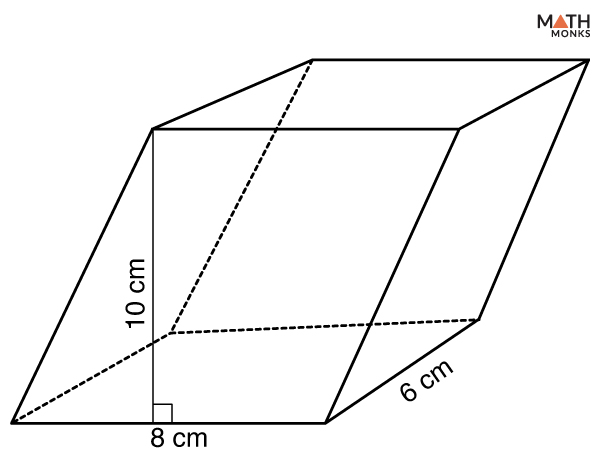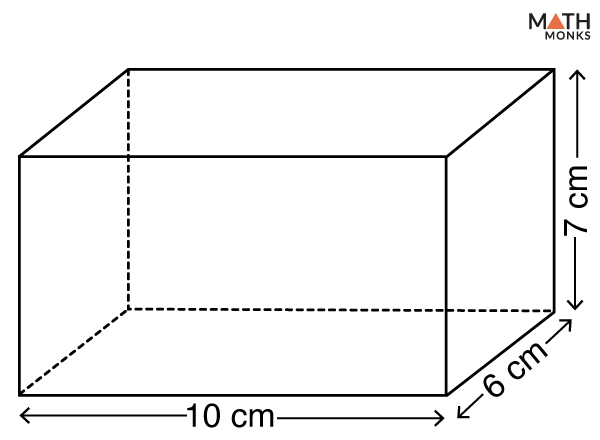# Parallelepiped

The word parallelepiped came from a Greek word meaning ‘an object having parallel plane’.

A Parallelepiped is thus a three-dimensional shape formed by six parallelogram-shaped faces. We can also define it as a polyhedron where three pairs of parallel faces join together to form a three-dimensional shape with six faces. It is referred to as a prism with a parallelogram-shaped base.

## Properties

1. Has 6 faces; in parallelepiped ABCDEFGH, ABCD, EFGH, EADH, FBCG, ABFE, and DCGH are the faces
2. Has 12 edges; AB, BC, CD, DA, EF, FG, GH, HE, EA, AB, BF, and FE are the edges
3. Has 8 vertices; A, B, C, D, E, F, G, and H are the vertices
4. All faces are in the shape of a parallelogram; ABCD, EFGH, EADH, FBCG, ABFE, and DCGH are parallelograms
5. Has 12 face diagonals (2 diagonals on each face); DG and CH are the face diagonals in the face DCGH. Similarly, there are 2 face diagonals for rest of the 5 faces
6. The diagonals connecting the vertices not lying on the same face are the body or space diagonals; EC and BH are the space diagonals
7. Each set of opposite faces are mirror images; ABCD and EFGH,  EADH and FBCG, and ABFE and DCGH are mirror images to each other

## Formulas

### Surface Area

The total surface area of a parallelepiped is the entire region covered by all its six faces or surfaces.

It is expressed in square units, like sq. m, sq. cm, sq. in, sq. ft, sq. yd, etc. A parallelepiped can have two types of surface areas:

1. Total Surface Area (TSA) – The total surface area of a parallelepiped is defined as the total region covered by all the faces of the shape.

2. Lateral Surface Area (LSA) – The lateral surface area of a parallelepiped is defined as the surface area of its faces excluding the base and the top. It is the product of the perimeter and the height of the parallelepiped.

The formulas are given below:

Let us solve an example to understand the concept better.Find the total surface area of the given parallelepiped.

Solution:

As we know,
Total Surface Area (TSA) = 2(ab + bc + ac), here a = 9 cm, b = 7 cm, c = 5 cm
= 2(9 × 7 + 7 × 5 + 9 × 5 )
= 286 sq. cmFind the lateral surface area of a parallelepiped with lateral edges 8 cm and 6 cm, and a height of 10 cm.

Solution:

As we know,
Lateral Surface Area (LSA) = 2(a + b) × h, here a = 8 cm, b = 6 cm, h = 10 cm
= 2 × (8 + 6) × 10
= 280 sq. cm

### Volume

The volume of a parallelepiped is defined as the total space occupied by it in a three-dimensional plane. It is expressed in cubic units such as cm3, m3, in3, ft3, yd3, etc.

Volume (V) = area of base ⋅ height

Using the formula for the cross product in component form, we can write the scalar triple product in component form as:

The formula is given below:

Let us solve an example to understand the concept better.Find the volume of parallelepiped with vectors a = (1, 1, 2), b = (2, 1, 3) and c = (3, 1, 2)

Solution:

As we know,
Volume (V) = |(a × b)⋅c|, here a = (1, 1, 2), b = (2, 1, 3) and c = (3, 1, 2)
|3           1             2|
|1           1             2|
|2           1             3|
= |3(1 × 3 – 2 × 1) – 1(1 × 3 – 2 × 2) + 2(1 × 1 – 1 × 2)|
= |3 × 1 – 1 × (-1) + 2 × (-1)|
= |2|
= 2 unit3

## Rectangular Parallelepiped

Rectangular parallelepiped, also popularly known as a cuboid, is a special parallelepiped whose all six faces are rectangular. The lengths of all the parallel edges are equal. A shoebox is a typical example of Rectangular parallelepiped.

### Surface Area

The total surface area of a rectangular parallelepiped is the total region covered by all its six faces. The formula to calculate the total surface area of a rectangular parallelepiped is given below:

Total Surface Area (TSA) = 2(ab + bc + ac), here a, b, and c are the three dimensions

### Volume

The volume of a rectangular parallelepiped is the total space occupied by it in a three-dimensional plane. The formula to calculate the volume of a rectangular parallelepiped is given below:

Volume (V) = a × b × c, here a, b, and c are the three dimensions

### Diagonal

The body or space diagonal of a rectangular parallelepiped is the line segment connecting the two vertices not lying on the same face. The formula to calculate the space diagonal of a rectangular parallelepiped is given below:

Diagonal (D) = √(a2 + b2 + c2) , here a, b, and c are the three dimensions

Finding the total surface area of a rectangular parallelepiped when the THREE DIMENSIONS are known

Find the amount of paper needed to wrap a gift box with lateral faces of 10 cm and 6 cm and height 7 cm.

Solution:

As we know, the amount of paper needed = the total surface area of the box,
Total Surface Area (TSA) = 2(ab + bc + ac), here a = 10 cm, b = 6 cm, c = 7 cm
= 2(10 × 6 + 6 × 7 + 7 × 10)
= 344 sq. cm

Finding the volume of a rectangular parallelepiped when the THREE DIMENSIONS are known

Find the volume of a rectangular parallelepiped whose dimensions are 11 cm, 8 cm and 5 cm respectively.

Solution:

As we know,
Volume (V) = w × l × h, here w = 8 cm, l = 11 cm, h = 5 cm
= 8 × 11 × 5
= 440 cm3

Finding the space diagonal of a rectangular parallelepiped when the THREE DIMENSIONS are known

Find the volume of a rectangular parallelepiped whose dimensions are 4 cm, 5 cm and 7 cm respectively.

Solution:

As we know,
Diagonal (D) = √(a2 + b2 + c2), here a = 4 cm, b = 5 cm, c = 7 cm
= √(4 × 4 + 5 × 5 + 7 × 7)
= √90
= 9.49 cm